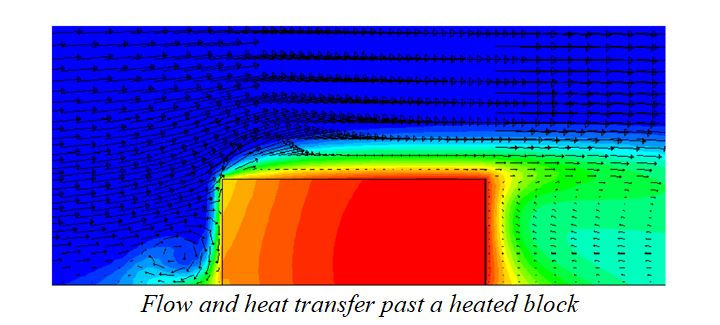top of page
Search
• manchestercfd

# ‘Convection’…the most common heat transfer mode in CFD!

Introduction

Convective heat transfer is said to occur when there is a transport of thermal energy by molecular conduction and bulk fluid motion and it is traditionally divided into the regimes of ‘forced’ convection (where motion is in response to an externally-applied pressure difference) and ‘natural’ convection (where motion is due to density variations within the fluid).

However, both mechanisms may operate simultaneously; where there is a buoyancy-modified forced flow, the heat transfer regime is termed ‘mixed’ (or ‘combined’) convection.

The effects on heat transfer performance are complex, and forced and free convection influences do not combine in a simple additive manner.

In convective heat transfer:

• Heat transfer rate can be closely coupled to the fluid flow solution.

• The rate of heat transfer is strongly dependent on fluid velocity and fluid properties.

• Fluid properties may vary significantly with temperature.

For example when cold air flows past a warm body, it draws away warm air near the body and replaces it with cold air.

Convection can be considered to be the most common and most important mode of heat transfer in CFD and has numerous applications in oil and gas, nuclear, renewable energy sector, amongst others.Newton’s law of cooling states that(101)Generally, there are four types of convection.

1) Natural Convection: Fluid moves due to buoyancy effects.

2) Forced Convection: Flow is induced by some external means.

3) Mixed Convection: When Natural and forced convection exist simultaneously.

4) Boiling Convection: Body is hot enough to cause fluid phase change.

In general, the heat transfer coefficient, h is not constant but is usually a function of temperature gradient.Natural Convection

In natural convection, fluid motion occurs due to buoyancy effects. As the fluid is heated, its density decreases. This density gradient causes a buoyant force opposite to be generated which induces flow to gravity.

The buoyancy force (per unit volume) can be computed byBoundary Layer Flow

Analogous to the viscous boundary layer that develops, there is also a thermal boundary layer.Heat Transfer Coefficient Correlations

Various correlations can be used to find the Nusselt number. For example, heat transfer resulting from flow around a sphere can be found by using:Similarly, heat transfer from a flat plate in laminar flow (thermal boundary layer) is calculated through:## Recent Posts

See All

1. Modes of Heat Transfer Conduction, convection and radiation are the three mechanisms by which thermal energy may be transferred from one point in space (and time) to another. • Conduction: diffusio

bottom of page